# Understanding quantum bit notation

## Turns out it’s somewhat intuitive!

• After a qubit collapses to |0or |1, it does not go back into superposition. If it collapsed to |0, it is now |0.

# Expressing 1 qubit

## Expanded notation for 1 qubit

Let’s say we have a qubit, |φ. Perhaps you have seen expanded notation:

• b² is the probability that |φ will collapse to |1when measured
• By similar logic, |0represents a qubit that always collapses to (or “has collapsed to”) |0.

## Vector notation for 1 qubit

There exists another way to express qubits, which involves vectors. If you’ve never seen vectors or matrices, you should check out this introduction.

• b² is still the probability that the qubit will collapse to |1 when measured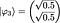Example #3. This is the vector notation of Example #1. (Fig 8)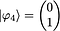Example #4. This is the vector notation of Example #2. (Fig 9)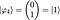Example #4: |φ₄⟩ has a 100% chance of collapsing to |1⟩ when measured, so it is |1⟩. (Fig 10)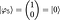Example #5. (Fig 11)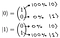Note, it just happens that (1)² = 100% and (0)² = 0%, so it seems the values weren’t squared. (Fig 12)
• (bottom number)² = probability the qubit collapses to |1when measured

# Expressing 2+ qubits

## Expanded notation for 2+ qubits

We can use expanded notation in a very similar manner. Note, though, that each additional qubit doubles the number of fundamental states:

## Vector notation for 2+ qubits

A multi-qubit system can also be represented as a vector. For simplicity, let’s consider a 2-qubit system (we write |00to |11in ascending binary order):

• qubit 2 (blue) in state |1
• y² = probability that qubit 1 collapses to |1 when measured
• z² = probability that qubit 2 collapses to |0 when measured
• w² = probability that qubit 2 collapses to |1 when measured
1. It is also x² z² since collapsing to |00 means:
• qubit 2 collapses to |0(with probability z²)
• x² w² (qubit 1 collapses to |0and qubit 2 collapses to |1)
• (3) compute the tensor product
• (4) rewrite in terms of a, b, c, d using the equations from Fig 24

# What next?

Understanding qubits in vector notation becomes very helpful when you begin to work with quantum logic gates. The gates can be represented as matrices so that their interactions with qubits become matrix multiplications. If you’re interested in how that works, check out this lecture about the mathematical representation of quantum computing.

Written by